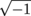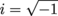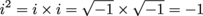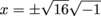Study Guide

# Quadratic Formula and Functions - Imaginary and Complex Numbers

## Imaginary and Complex Numbers

It's finally come to this, has it? We've run out of actual numbers to throw at you, so now we're just going to make some numbers up? Not really. Imaginary numbers, despite the name, are totally legitimate numbers in their own right. It's just that all of the numbers we've worked with so far are the real numbers, and what else are you going to call something that isn't "real?" Calling them fake numbers wouldn't really help things.

Okay, so what are imaginary numbers? We'll answer that with another question: what is? It should be a number that, when squared, equals -1, but any positive or negative real number will be positive when squared. We define a new type of number, called i, and state that. You can see that i2 = -1, just like we wanted.

### Sample Problem

What are the first 8 powers of i equal to? That is, i1, i2, i3 and so on up to i8

We already know that i1 = i and i2 = –1. So the rest are:

i3 = i i2 = i • -1 = -i

i4 = i i3 = i • -i = -i2 = -(-1) = 1

i5 = i i4 =

i6 = i i5 = i i = i2 = -1

i7 = i i6 = i • -1 = -i

i8 = i i7 = i • -i = -i2 = -(-1) = 1

As you've probably guessed, all powers of i can be expressed in terms of just the first four powers: i, -1, -i, and 1.

### Sample Problem

Solve x2 = -16.

It used to be that we'd see a problem like this, throw up our hands, say, "There's no real solution. There's nothing we can do!" and call it quits. Now that we know about i and imaginary numbers, this problem is almost too easy.x = ±4i

Then we double check our solutions by plugging 'em back into the original equation.

(4i)2 = 16i2 = -16

(-4i)2 = (-4)2i2 = 16i2 = -16

Check and check.

Complex numbers are pretty simple. They all take the form a + bi, where a and b are real numbers. So, they have both a real and imaginary component, like a baseball game between the Yankees and the X-men. Addition, subtraction, and multiplication all work pretty much how you would expect them to.

(-1 + 2i) + (3 – i) = 2 + i

(-1 + 2i) – (3 – i) = -4 + 3i

(-1 + 2i)(3 – i) = -3 + i + 6i – 2i2 = -3 + 7i – 2(-1) = -1 + 7i

The imaginary term is handled just like you would another variable.

Division with complex numbers is different from what you'd expect. We'll cover that another time, though, when we aren't so sleepy.